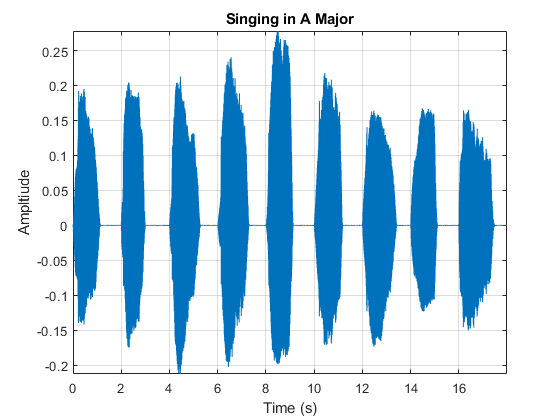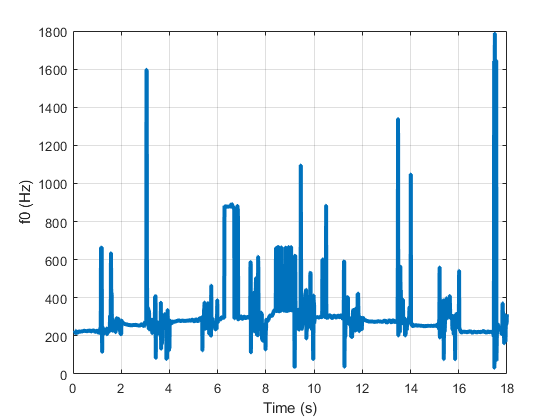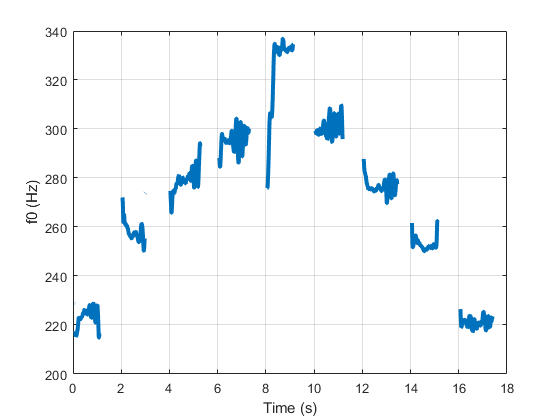# pitchnn

Estimate pitch with deep learning neural network

## Syntax

``f0 = pitchnn(audioIn,fs)``
``f0 = pitchnn(audioIn,fs,Name,Value)``
``[f0,loc] = pitchnn(___)``
``[f0,loc,activations] = pitchnn(___)``
``pitchnn(___)``

## Description

example

````f0 = pitchnn(audioIn,fs)` returns estimates of the fundamental frequency over time for `audioIn` with sample rate `fs`. Columns of the input are treated as individual channels.```
````f0 = pitchnn(audioIn,fs,Name,Value)` specifies options using one or more `Name,Value` arguments. For example, `f0 = pitchnn(audioIn,fs,'ConfidenceThreshold',0.5)` sets the confidence threshold for each value of `f0` to `0.5`.```
````[f0,loc] = pitchnn(___)` returns the time values, `loc`, associated with each fundamental frequency estimate.```
````[f0,loc,activations] = pitchnn(___)` returns the activations of a `crepe` pretrained network.```
````pitchnn(___)` with no output arguments plots the estimated fundamental frequency over time.```

## Examples

collapse all

Type `crepe` at the Command Window. If the Audio Toolbox model for CREPE is not installed, then the function provides a link to the location of the network weights. To download the model, click the link and unzip the file to a location on the MATLAB path.

```downloadFolder = fullfile(tempdir,'crepeDownload'); loc = websave(downloadFolder,'https://ssd.mathworks.com/supportfiles/audio/crepe.zip'); crepeLocation = tempdir; unzip(loc,crepeLocation) addpath(fullfile(crepeLocation,'crepe'))```

Check that the installation is successful by typing `crepe` at the Command Window. If the network is installed, then the function returns a `DAGNetwork` (Deep Learning Toolbox) object.

`crepe`
```ans = DAGNetwork with properties: Layers: [34×1 nnet.cnn.layer.Layer] Connections: [33×2 table] InputNames: {'input'} OutputNames: {'pitch'} ```

The CREPE network requires you to preprocess your audio signals to generate buffered, overlapped, and normalized audio frames that can be used as input to the network. This example demonstrates the `pitchnn` function performing all of these steps for you.

Read in an audio signal for pitch estimation. Visualize and listen to the audio. There are nine vocal utterances in the audio clip.

```[audioIn,fs] = audioread('SingingAMajor-16-mono-18secs.ogg'); soundsc(audioIn,fs) T = 1/fs; t = 0:T:(length(audioIn)*T) - T; plot(t,audioIn); grid on axis tight xlabel('Time (s)') ylabel('Ampltiude') title('Singing in A Major')```Use the `pitchnn` function to produce the pitch estimate using a CREPE network with `ModelCapacity` set to `tiny` and `ConfidenceThreshold` disabled. Calling `pitchnn` with no output arguments plots the pitch estimation over time. If you call `pitchnn` before downloading the model, an error is printed to the Command Window with a download link.

`pitchnn(audioIn,fs,'ModelCapacity','tiny','ConfidenceThreshold',0)`With confidence thresholding disabled, `pitchnn` provides a pitch estimate for every frame. Increase the `ConfidenceThreshold` to `0.8`.

`pitchnn(audioIn,fs,'ModelCapacity','tiny','ConfidenceThreshold',0.8)`Call `pitchnn` with `ModelCapacity` set to `full`. There are nine primary pitch estimation groupings, each group corresponding with one of the nine vocal utterances.

`pitchnn(audioIn,fs,'ModelCapacity','full','ConfidenceThreshold',0.8)`Call `spectrogram` and compare the frequency content of the signal with the pitch estimates from `pitchnn`. Use a frame size of `250` samples and an overlap of `225` samples or 90%. Use `4096` DFT points for the transform.

`spectrogram(audioIn,250,225,4096,fs,'yaxis')`## Input Arguments

collapse all

Input signal, specified as a column vector or matrix. If you specify a matrix, `pitchnn` treats the columns of the matrix as individual audio channels.

Data Types: `single` | `double`

Sample rate of the input signal in Hz, specified as a positive scalar.

Data Types: `single` | `double`

### Name-Value Arguments

Specify optional comma-separated pairs of `Name,Value` arguments. `Name` is the argument name and `Value` is the corresponding value. `Name` must appear inside quotes. You can specify several name and value pair arguments in any order as `Name1,Value1,...,NameN,ValueN`.

Example: `pitchnn(audioIn,fs,'OverlapPercentage',50)` sets the percent overlap between consecutive audio frames to 50.

Percentage overlap between consecutive audio frames, specified as a scalar in the range [0,100).

Data Types: `single` | `double`

Confidence threshold for each value of `f0`, specified as a scalar in the range [0,1).

To disable threshold, set this argument to `0`.

Note

If the maximum value of the corresponding `activations` vector is less than `'ConfidenceThreshold'`, `f0` is `NaN`.

Data Types: `single` | `double`

Model capacity, specified as `'tiny'`, `'small'`, `'medium'`, `'large'`, or `'full'`.

Tip

`'ModelCapacity'` controls the complexity of the underlying deep learning neural network. The higher the model capacity, the greater the number of nodes and layers in the model.

Data Types: `string` | `char`

## Output Arguments

collapse all

Estimated fundamental frequency in Hertz, returned as an N-by-C array, where N is the number of fundamental frequency estimates and C is the number of channels in `audioIn`.

Data Types: `single`

Time values associated with each `f0` estimate, returned as a `1`-by-N vector, where N is the number of fundamental frequency estimates. The time values correspond to the most recent samples used to compute the estimates.

Data Types: `single` | `double`

Activations from the CREPE network, returned as an N-by-`360`-by-C matrix, where N is the number of generated frames from the network and C is the number of channels in `audioIn`.

Data Types: `single` | `double`

 Kim, Jong Wook, Justin Salamon, Peter Li, and Juan Pablo Bello. “Crepe: A Convolutional Representation for Pitch Estimation.” In 2018 IEEE International Conference on Acoustics, Speech and Signal Processing (ICASSP), 161–65. Calgary, AB: IEEE, 2018. https://doi.org/10.1109/ICASSP.2018.8461329.

## Support#### Try MATLAB, Simulink, and Other Products

Get trial now